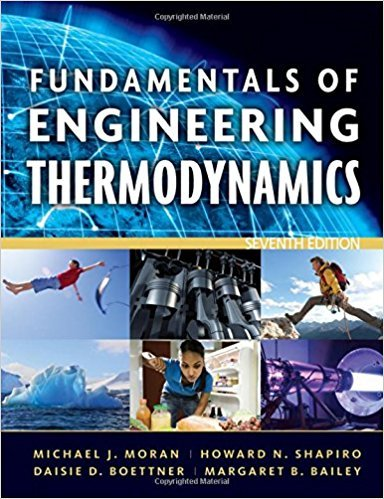×
Get Full Access to Fundamentals Of Engineering Thermodynamics - 7 Edition - Chapter 13 - Problem 113p
Get Full Access to Fundamentals Of Engineering Thermodynamics - 7 Edition - Chapter 13 - Problem 113p

×

# Exergy Analysis of Reacting and Psychrometric SystemsUsingISBN: 9780470495902 50

## Solution for problem 113P Chapter 13

Fundamentals of Engineering Thermodynamics | 7th Edition

• Textbook Solutions
• 2901 Step-by-step solutions solved by professors and subject experts
• Get 24/7 help from StudySoup virtual teaching assistantsFundamentals of Engineering Thermodynamics | 7th Edition

4 5 1 405 Reviews
21
3
Problem 113P

PROBLEM 113P

Exergy Analysis of Reacting and Psychrometric Systems

Using the result of Problem 13.111(b), determine the total specific flow exergy at locations 1, 2, and 3 and the rate of exergy destruction, each in Btu/min, for the device of Problem 12.102. Let the environment be a mixture of dry air and water vapor at 95°F, 1 atm withAlso, let cpa = 0.24 Btu/lb · °R and cpv = 0.44 Btu/lb · °R

Problem 12.102

Figure P12.102 shows the adiabatic mixing of two moist-air streams at steady state. Kinetic and potential energy effects are negligible. Determine the rate of exergy destruction, in Btu/min, for T0 = 95°F.Fig. P12.102

Problem 13.111(b)

Exergy Analysis of Reacting and Psychrometric Systems

For psychrometric applications such as those considered in Chap. 12, the environment often can be modeled simply as an ideal gas mixture of water vapor and dry air at temperature T0 and pressure p0. The composition of the environment is defined by the dry air and water vapor mole fractions,, respectively.

(b) Express the result of part (a) on a per unit mass of dry air basis aswhereandStep-by-Step Solution:
Step 1 of 3

Week 11: Air Quality 11.8.16 Composition ● Nitrogen (76%) Air Quality ● Many European cities do not meet world health organization air quality standards for at least one pollutant ● In the US, about a quarter of the population lives in areas that do not meet us air quality standards Smog ● A mixture of pollutants, principally ground-level ozone, produced by chemical reactions in the air involving smog-forming chemicals ● Formed by anthropogenic and/or natural sources ● Enters body through inhalation. Ozone is highly reactive (O3) reacts in your airways. Causes issues for asthmatics Temperature inversion ● An atmospheric condition during which a warm layer of air stalls above a layer of cool air that is closer

Step 2 of 3

Step 3 of 3

##### ISBN: 9780470495902

This full solution covers the following key subjects: air, exergy, Dry, btu, psychrometric. This expansive textbook survival guide covers 14 chapters, and 1501 solutions. The full step-by-step solution to problem: 113P from chapter: 13 was answered by , our top Engineering and Tech solution expert on 07/20/17, 09:01AM. The answer to “Exergy Analysis of Reacting and Psychrometric SystemsUsing the result of 13.111(b), determine the total specific flow exergy at locations 1, 2, and 3 and the rate of exergy destruction, each in Btu/min, for the device of 12.102. Let the environment be a mixture of dry air and water vapor at 95°F, 1 atm with Also, let cpa = 0.24 Btu/lb · °R and cpv = 0.44 Btu/lb · °R 12.102Figure P12.102 shows the adiabatic mixing of two moist-air streams at steady state. Kinetic and potential energy effects are negligible. Determine the rate of exergy destruction, in Btu/min, for T0 = 95°F. Fig. P12.102 13.111(b)Exergy Analysis of Reacting and Psychrometric SystemsFor psychrometric applications such as those considered in Chap. 12, the environment often can be modeled simply as an ideal gas mixture of water vapor and dry air at temperature T0 and pressure p0. The composition of the environment is defined by the dry air and water vapor mole fractions , , respectively.(b) Express the result of part (a) on a per unit mass of dry air basis as where and” is broken down into a number of easy to follow steps, and 180 words. Since the solution to 113P from 13 chapter was answered, more than 267 students have viewed the full step-by-step answer. Fundamentals of Engineering Thermodynamics was written by and is associated to the ISBN: 9780470495902. This textbook survival guide was created for the textbook: Fundamentals of Engineering Thermodynamics, edition: 7.

Unlock Textbook Solution